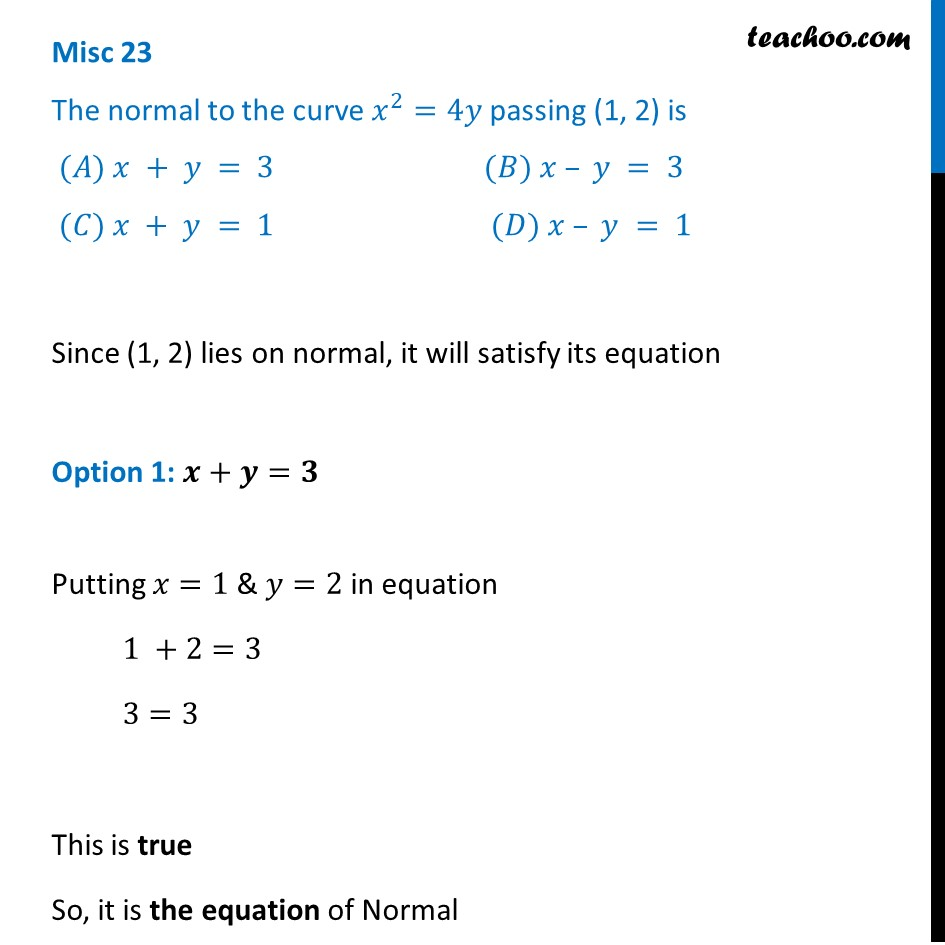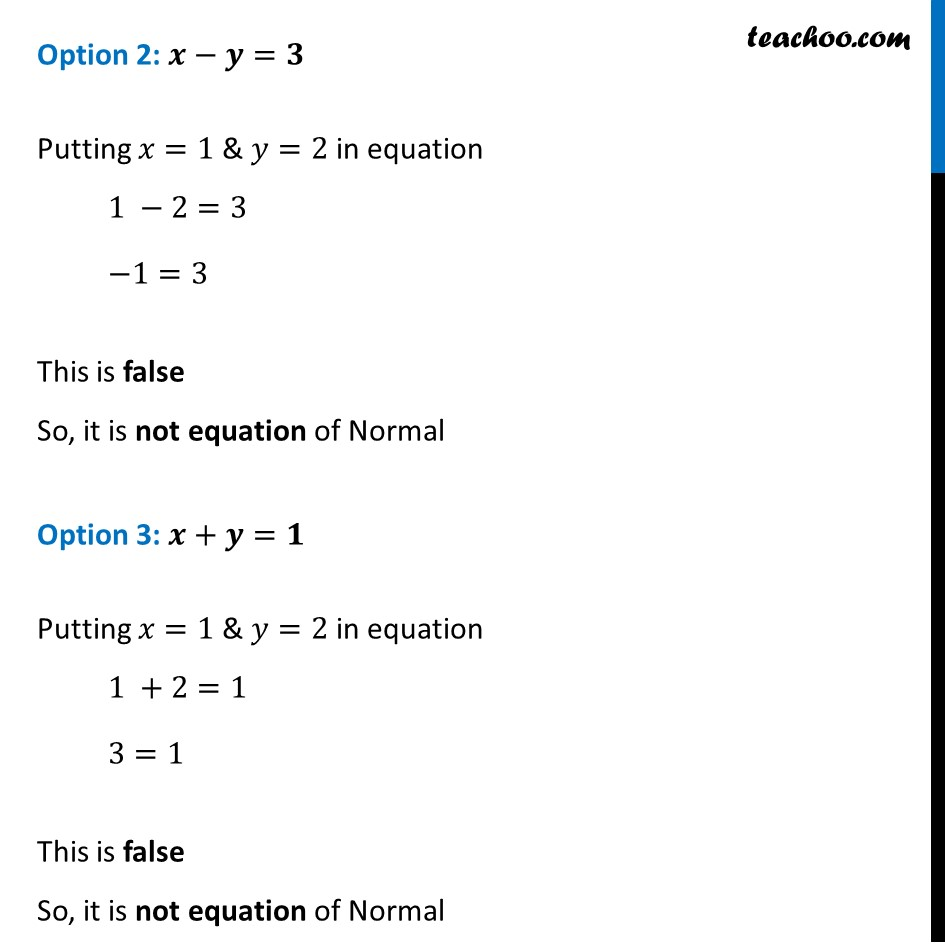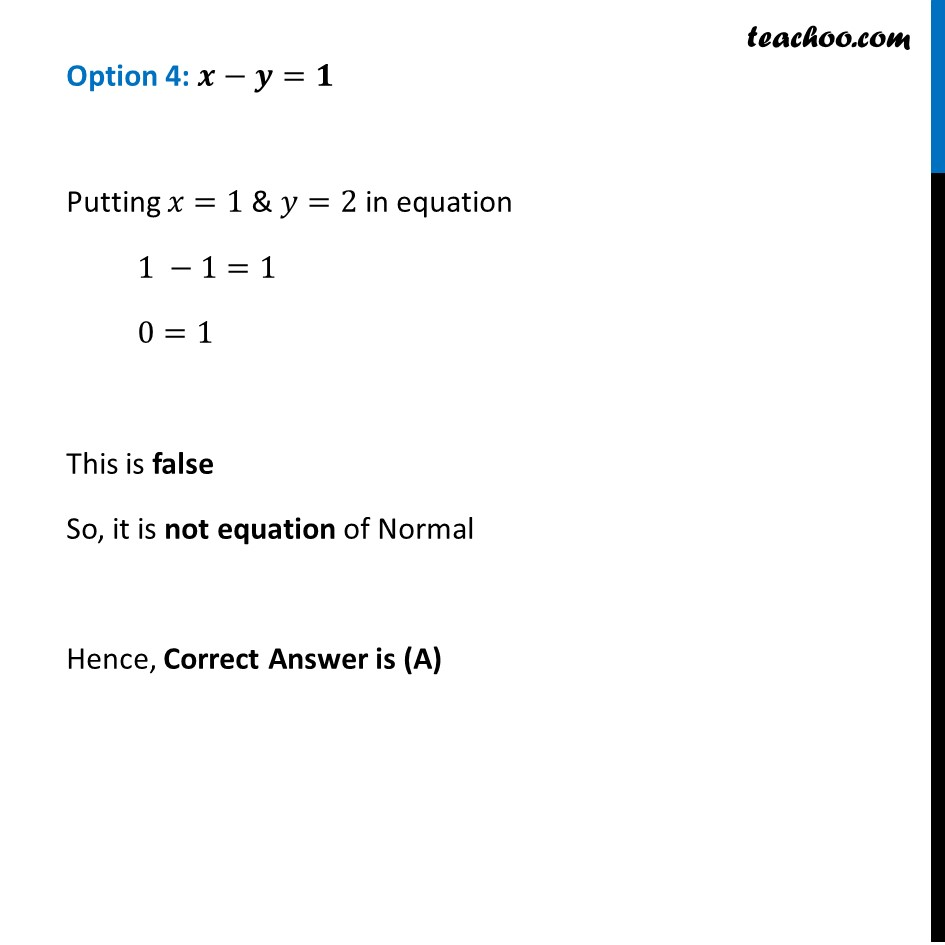Finding equation of tangent/normal when point and curve is given

Chapter 6 Class 12 Application of Derivatives
Concept wiseLearn in your speed, with individual attention - Teachoo Maths 1-on-1 Class

### Transcript

Misc 23 The normal to the curve 𝑥^2=4𝑦 passing (1, 2) is (𝐴) 𝑥 + 𝑦 = 3 (𝐵) 𝑥 – 𝑦 = 3 (𝐶) 𝑥 + 𝑦 = 1 (𝐷) 𝑥 – 𝑦 = 1Since (1, 2) lies on normal, it will satisfy its equation Option 1: 𝒙+𝒚=𝟑 Putting 𝑥=1 & 𝑦=2 in equation 1 +2=3 3=3 This is true So, it is the equation of Normal Option 2: 𝒙−𝒚=𝟑 Putting 𝑥=1 & 𝑦=2 in equation 1 −2=3 −1=3 This is false So, it is not equation of Normal Option 3: 𝒙+𝒚=𝟏 Putting 𝑥=1 & 𝑦=2 in equation 1 +2=1 3=1 This is false So, it is not equation of Normal Option 4: 𝒙−𝒚=𝟏 Putting 𝑥=1 & 𝑦=2 in equation 1 −1=1 0=1 This is false So, it is not equation of Normal Hence, Correct Answer is (A)